2022: SklogWiki celebrates 15 years on-line

# Nosé-Hoover thermostat

The Nosé-Hoover thermostat   is a method for controlling the temperature in a molecular dynamics simulation. The Nosé-Hoover thermostat "strives" to reproduce the canonical phase-space distribution. It does this by modifying the equations of motion to include a non-Newtonian term in order to maintain the total kinetic energy constant. The modified equation of motion is given by (Ref. 3 Eq. 4)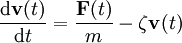$\frac{{\mathrm {d}}{\mathbf{v}}(t)}{{\mathrm {d}t}} = \frac{{\mathbf {F}}(t)}{m} -\zeta {\mathbf{v}}(t)$

where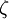$\zeta$ is the thermodynamic friction coefficient, given by (Ref. 3 Eq. 5)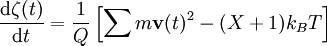$\frac{{\mathrm {d}}\zeta(t)}{{\mathrm {d}t}} = \frac{1}{Q} \left[ \sum m {\mathbf{v}}(t)^2 - (X+1)k_BT \right]$

where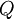$Q$ is a parameter that has the dimensions of energy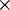$\times$(time)2 and determines the time-scale of the temperature fluctuation and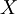$X$ is the number of degrees of freedom.

## Problems

The Nosé-Hoover thermostat has problems with ergodicity for small or stiff systems. In order to compensate for this a modification using "chains" has been proposed .

## Non-equilibrium

A version of the Nosé-Hoover thermostat has been developed for non-equilibrium simulations .